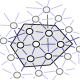9:08 AM
0I recently created a new tag: mathematical-german. Other than English, the main languages in which mathematics is published are French, German and Russian. We have a tag mathematical-french already. Thus, why not have a tag for German, too? One could post on the German Language SE, but there are...

2 hours later…11:17 AM
As you can see above, a new tag was created by Rodrigo de Azevedo.
6How do you express the common phrases "by an abuse of notation", "abusing the notation", etc. in German without invoking negative connotations? (Without this addendum, I'd have asked at german.se.) "Durch/Unter Missbrauch der Schreibweise/Notation" looks horrible to me, in particular due to sexua...

2How to translate the standard mathematical mantra "By an abuse of terminology, …" into German? E.g. An environment is a family of partial maps $\eta_M\colon X_M\dashrightarrow\dots$ for $M\in S$ such that … By an abuse of terminology, if the sets $X_M$ for $M\in S$ are pairwise disjoint...

0In a recent publication called "Secure passwords using combinatorial group theory," by G. Baumslag et al., it says that the following book is a "standard reference" in combinatorial group theory. Camps, Gr Rebel and Rosenberger, "Einführung in die Kombinatorische und die Geometrische Gruppentheo...

1I tried translating the word "beschränkt" and then google, for example, "limited set", but got no results.

2Where could I find either a translation or an account of van der Waerden's arguments in his 1936 paper Die Seltenheit der reduziblen Gleichungen und der Gleichungen mit Affekt?

1In almost any German book on the theory of functions the term "Nebenteil" is used for the part of a Laurent series which has non-negative exponents. E. g., if the Laurent series $\sum_{\nu = -\infty }^\infty a_\nu z^\nu$ is given, the "Nebenteil" would be $$\sum_{\nu = 0}^\infty a_\nu z^\nu.$$ I...

2In complex path integration there is the following fundamental inequality $$\left\vert \int_\gamma f(z) dz \right\vert \leq \mathcal{L} \left( \gamma\right) \cdot \max \left\lbrace\left\vert f \left( z \right) \right\vert : z \in \gamma^*\right\rbrace$$ where $\mathcal{L} \left( \gamma\right... 1Für jede natürliche Zahl$n \in \mathbb{N}$sei$A_n$die von der Mengenfamilie$\{\{1\}, \{2\}, \dots , \{n\}\}$erzeugte sigma-algebra auf$\mathbb{N}$. Zeigen Sie, daß$A_n$neben$B = \emptyset$und$B = \mathbb{N}$aus allen Mengen$A \subset \mathbb{N}$besteht, welche entweder$A \subse...

1In German, "Grundbereich" and "Träger" stand for the set over which a structure is defined. For example, for the structure $G=(\mathbb{R},\cdot,1)$ the "Grundbereich" or "Träger" is $\mathbb{R}$.

7 hours later…6:30 PM
@MartinSleziak partly my fault I suppose. I had seen your ping but was slow to act. sorry.